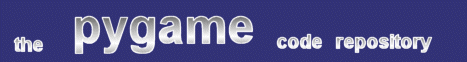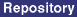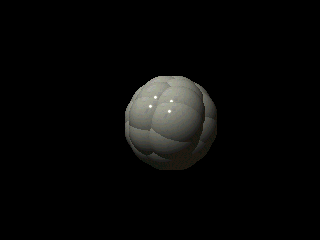### Title: Intersection Bobs

Author: Diez B. Roggisch (deets at web.de)
Submission date: September 22, 2002

Description: Simple intersection bob example, plus usage of invalidate rects to restore background.pygame version required: Any with Surfarray
SDL version required: Any
Python version required: 2.0 with Numeric

Comments: A demo effect from the early 90ties, intersection bobs combined vector bobs with precalculated z-buffers. The results can be very impressive. A extension could be to use an animated bob with a different shape than a sphere.

The archive file includes the POV files used to create the sphere used for the bob source graphics.

```
"""
A demo effect from the early 90ties, intersection bobs combined vector bobs with
precalculated z-buffers. The results can be very impressive. A extension could be to
use a animated bob with a different shape than a sphere.
"""

try:
import sys, types, pygame, pygame.image, pygame.display, pygame.rect, pygame.key
import profile
from Numeric import *

except ImportError, e:
print "import exception occured"
print e
sys.exit()

MODE = (640, 480)
BACKGROUND = None
BALL = None

class ZSprite:
""" A ZSprite combines a surface and a hight-mask. The latter one should be supplied as
a grey-image with values from 0..255. 0 means that the sprite doesn't use that pixel at all,
255 is a pixel with the mininal z coordinate (aka closest to the viewer).
"""

# The bias is used to ease calculations. This insures that the
# crucial zero never appears as coordinate.
BIAS = 2 ** 24
def __init__(_, surface, z_surface):
if surface.get_size() != z_surface.get_size():
raise "surface and z_surface dimensions differ!"
_.surface = surface
_.createZArray(z_surface)

def createZArray(_, z_surface):
_.z_array = asarray(pygame.surfarray.array3d(z_surface)[:,:,1], Int32)
_.z_mask = greater(_.z_array, 0)

return ((_.z_array + z) + ZSprite.BIAS) * _.z_mask

def __sub__(_, z):
return ((_.z_array - z) + ZSprite.BIAS) * _.z_mask

class BobObject:
""" A BobObject. The list _.coords are the coordinates of the points to be blitted """
def __init__(_, zsprite, scadjust):
""" surface - the points are blitted as that
scadjust - a tuple representing the screen (width/2, height/2) to center the transformed coordinates
"""
_.surface = zsprite.surface
_.zsprite = zsprite
_.coords = []
_.scadjust = scadjust - array(_.surface.get_rect().size) / 2
_.position = array((0,0,0))
_.reset()
_.colObs = []

def translate(_, trans):
_.position += trans

def reset(_):
_.orientation = array(identity(3), Float)

def rotateZ(_, deg):
rMat = array(identity(3), Float)
cos = math.cos(deg)
sin = math.sin(deg)
rMat = cos
rMat = -sin
rMat = sin
rMat = cos
_.orientation = matrixmultiply(_.orientation, rMat)

def rotateY(_, deg):
rMat = array(identity(3), Float)
cos = math.cos(deg)
sin = math.sin(deg)
rMat = cos
rMat = -sin
rMat = sin
rMat = cos
_.orientation = matrixmultiply(_.orientation, rMat)
_.rot = deg

def rotateX(_, deg):
rMat = array(identity(3), Float)
cos = math.cos(deg)
sin = math.sin(deg)
rMat = cos
rMat = -sin
rMat = sin
rMat = cos
_.orientation = matrixmultiply(_.orientation, rMat)

def screenCoords(_):
""" This methods transforms and projects the coordinates of the bobs.
"""
sc = []
_.colObs = []
for c in _.coords:
p = matrixmultiply(c , _.orientation) + _.position
sc.append((p, int(p * 1000 / p + _.scadjust), int(p * 1000 / p + _.scadjust), int(p)))

#sc.sort()
#sc.reverse()
sc = map(lambda x: x[1:], sc)
left = reduce(lambda x,y:(y,x)[x < y], sc)
right = reduce(lambda x,y:(y,x)[x > y], sc)
top = reduce(lambda x,y:(y,x)[x < y], sc)
bottom = reduce(lambda x,y:(y,x)[x > y], sc)
size = (int(right - left), int(bottom - top))
# The transformed screencoords
_.screen_coords = sc
# The extend of all sprites together.
_.size = size
_.topleft = (left, top)

def calcSurfaces(_):
""" This is the primary method: here we calculate the individual masks for the bobs.
"""
_.surfaces = []
# The back buffer is zero-initialized
ssz =_.zsprite.surface.get_size()
back = zeros((_.size + 16 + ssz, _.size + 16 + ssz))
for i in xrange(len(_.coords)):
# tl is the coordinate of the bob relative to the
# back buffer
tl = _.screen_coords[i] - _.topleft + 8, _.screen_coords[i] - _.topleft + 8
sz = _.zsprite.surface.get_size()
br = tl + sz, tl + sz
# Get the zmap for the child
zmap = _.zsprite - _.screen_coords[i]
s1 = slice(tl,br)
s2 = slice(tl,br)
# The part of the back this sprite is going to cover
portion = back[s1, s2]
diff = zmap - portion
# Every pixel where the current bob has a smaller z coordinate
# will be set by it, the rest is cleared.
mask = asarray(greater(diff, 0), Int32)

# Propagate the values in the back map
back[s1, s2] = (portion + (diff * mask)).astype(Int32)
# This makes the mask a valid alpha channel
# Create a new surface
sf = _.zsprite.surface.convert_alpha()
sa = pygame.surfarray.pixels_alpha(sf)
# Apply the mask
_.surfaces.append(sf)

def getBobs(_):
""" Combine coordinates and computed images
"""
_.screenCoords()
_.calcSurfaces()
bobs = []
for i in xrange(len(_.coords)):
bobs.append((_.screen_coords[i][:-1], _.surfaces[i]))
return bobs

class Sphere(BobObject):
def __init__(_, dim, surface, scad, segments = 8):
nums = [(1, 0)]
for n in xrange(1, segments >> 1):
deg = n / float(segments >> 1) * math.pi / 2
radius = dim * cos(deg)
offset = dim * sin(deg)
num = floor(segments * cos(deg))
if int(num) & 1:
num += 1
for i in xrange(num):
x = radius * cos(math.pi * i / num * 2)
y = radius * sin(math.pi * i / num * 2)
_.coords.append((x, y, offset))
_.coords.append((x, y, -offset))

for i in xrange(segments):
x = dim * cos(math.pi * i / segments * 2)
y = dim * sin(math.pi * i / segments * 2)
_.coords.append((x, y, 0))
_.coords.append((0, 0, -dim))
_.coords.append((0, 0, dim))

class Ring(BobObject):
def __init__(_, dim, surface, scad, segments = 8):
for i in xrange(segments):
x = dim * cos(math.pi * i / segments * 2)
y = dim * sin(math.pi * i / segments * 2)
_.coords.append((x, y, 0))

class DisplayList:
def __init__(_):
_.saveStack = []
_.bobs = []
_.display = pygame.display.get_surface()

# Create the rect in the display which has to be saved
rect = bob.get_rect().move(bob)
bgSave = pygame.Surface(rect[2:])
bgSave.blit(_.display, (0,0), rect)
_.saveStack.append((bob, bgSave, rect))
_.bobs.append(bob)

def blitBobs(_):
rectList = []
for bob in _.bobs:
rectList.append(bob.get_rect().move(bob))
_.display.blit(bob, bob)
_.bobs = []
return rectList

def restoreBackground(_):
rectList = []
count = 0
y = 48
while len(_.saveStack):
resBob = _.saveStack.pop()
rectList.append(resBob)
_.display.blit(resBob, resBob)
count += 1
return rectList

def main():
global DISPLAY
global SCREENRECT
global LEVEL
global DIST_DEC
DIST_DEC = 1
pygame.init()
pygame.display.init()

rect = (0, 0) + MODE
DISPLAY = pygame.display.set_mode(MODE, 0, 32)
SPHERE = ZSprite(sphere_image, pygame.image.load("spherePP.png"))

dl = DisplayList()
sc = array(DISPLAY.get_rect()[2:])*0.5

vBob = Sphere(200, SPHERE, sc, 6)
vBob.translate(array((0, 0, 10009)))

pygame.display.update()
oldts = pygame.time.get_ticks() -100
speed = 50.0
at = 0
r = 1.0

while 1==1:
ts = pygame.time.get_ticks()
elapsed = ts - oldts
oldts = ts
pygame.event.pump()
keystate = pygame.key.get_pressed()
if keystate[pygame.K_ESCAPE]:
break

invalidRects = dl.restoreBackground()
vBob.reset()
r = r + speed * elapsed / 1000
vBob.rotateX((r / 180.0) * math.pi)
vBob.rotateY((r / 180.0) * math.pi)

bobs = vBob.getBobs()
for b in bobs:
invalidRects += dl.blitBobs()
pygame.display.update(invalidRects)
pygame.time.wait(50)

pygame.display.update()
pygame.time.wait(50)

if __name__ == "__main__":
main()
#profile.run('main()')

```
 From: deets - author Date: October 09, 2002 19:26 GMT After submitting the effect, I played around with it a little bit more. The effect looks more impressive if you alter the following two lines: in ZSprite, change _.z_array = asarray(pygame.surfarray.array3d(z_surface)[:,:,1], Int32) to _.z_array = asarray(pygame.surfarray.array3d(z_surface)[:,:,1], Int32) * 2 And in the main program vBob = Sphere(200, SPHERE, sc, 6) to vBob = Sphere(400, SPHERE, sc, 6) Play around with these values a little bit to create some nice effects. deets

 Feedback